Courses

# NCERT Solutions(Part- 3)- Mensuration Class 8 Notes | EduRev

## Class 8 : NCERT Solutions(Part- 3)- Mensuration Class 8 Notes | EduRev

The document NCERT Solutions(Part- 3)- Mensuration Class 8 Notes | EduRev is a part of the Class 8 Course Class 8 Mathematics by VP Classes.
All you need of Class 8 at this link: Class 8

Exercise 11.2

Question 1. The shape of the top surface of a table is a trapezium. Find its area it its parallel sides are 1 m and 1.2 m and perpendicular distance between them is 0.8 m.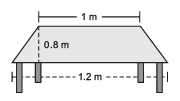Solution: ∵ Area of a trapezium = 1/2 * [Sum of parallel sides] * [Perpendicular distance between the parallel sides]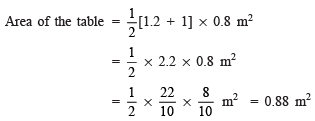Question 2. The area of a trapezium is 34 cm2 and the length of one of the parallel sides is 10 cm and its height is 4 cm. Find the length of the other parallel side.

Solution: Let the required other side = x cm

Area of the trapezium = 1/2 * [Sum of parallel sides ] * [Height]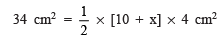or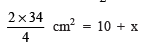or 10 + x = 17 ⇒ x = 17 – 10 = 7 cm

Hence, the required other side = 7 cm.

Question 3. Length of the fence of a trapezium shaped field ABCD is 120 m. If BC = 48 m, CD = 17 m and AD = 40 m, find the area of this field. Side AB is perpendicular to the parallel sides AD and BC.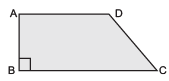Solution: Length of the fence = Perimeter

∴  AB + BC + CD + DA = 120

or AB + 48 + 17 + 40 = 120

or AB + 105 = 120

or AB = 120 – 105 = 15 m

∵ Area of a trapezium = 1/2 * [Sum of parallel sides] * Height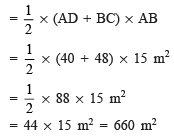Question 4. The diagonal of a quadrilateral shaped field is 24 m and the perpendiculars dropped on it from the remaining opposite vertices are 8 m and 13 m. Find the area of the field.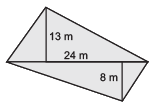= 1/2 * Diagonal * [Sum of the perpendiculars on the diagonal from opposite vertices]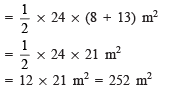Question 5. The diagonals of a rhombus are 7.5 cm and 12 cm. Find its area.

Solution: Area of the rhombus = 1/2 *Product of diagonals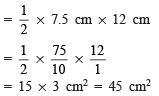Question 6. Find the area of a rhombus whose side is 6 cm and whose altitude is 4 cm. If one of its diagonals is 8 cm long, find the length of the other diagonal.

Solution: A rhombus is a parallelogram.

Area of a parallelogram = Base * Height

∴ Area of the rhombus = Base * Height

= 6 * 4 cm2 = 24 cm2

Let the other diagonal be d.

∴ Area of the rhombus = 1/2 * 8 * d = 4d [∵ One of the diagonals = 8 cm]

or 4d = 24

or d = 24/4 = 6 cm

Thus, the required other diagonal is 6 cm.

Question 7. The floor of a building consists of 3000 tiles which are rhombus shaped and each of its diagonals are 45 cm and 30 cm in length. Find the total cost of polishing the floor, if the cost per m2 is Rs 4.

Solution: Tiles are rhombus shaped, having d1 = 45 cm and d2 = 30 cm.

∴ Area of a tile (rhombus) = 1/2 * d1 * d2

= 1/2 * 45 * 30 cm2

= 45 * 15 cm2 = 675 cm2

Total number of tiles = 3000

∴ Area of the floor = 675 * 3000 cm2

= 2025000 cm2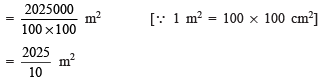Cost of polishing the floor = Rs 4 * 2025/10

= Rs 2 * 405 = Rs 810

Question 8. Mohan wants to buy a trapezium shaped field. Its side along the river is parallel to and twice the side along road. If the area of this field is 10500 m2 and the perpendicular distance between the two parallel sides is 100 m, find the length of the side along the river.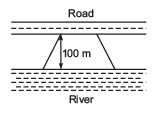Solution: Let the length of the side along the river = x m

∴ Other side (parallel to the road) = 1/2 xm

∴  Area of the trapezium shaped field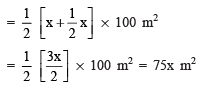But area of the field = 10500 m2

∴ 75x m2 = 10500 m2

or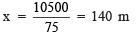Thus, length of the side along the river = 140 m

Question 9. Top surface of a raised platform is in the shape of regular octagon as shown in the figure. Find the area of the octagonal surface.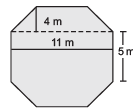Solution: This regular octagon can be into two trapeziums (each having height 4 m and parallel sides as 11 m and 5 m) and a rectangular part with length 11 m and breadth 5 m.

Area of a trapezium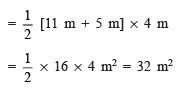∴  Area of both the trapeziums = 2 * 32 m2 = 64 m2

Now, Area of the rectangular part = l * b

= 11 m * 5 m = 55 m2

∴ Area of the regular octagon = 64 m2 + 55 m2 = 119 m2

Question 10. There is a pentagonal shaped park as shown in the figure. For finding its area Jyoti and Kavita divided it in two different ways.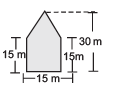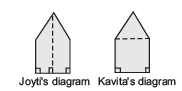Find the area of this park using both ways. Can you suggest some other way of finding its area?

Solution: Jyoti’s diagram:

The given shape is splitted into two congruent trapeziums.

Area of one trapezium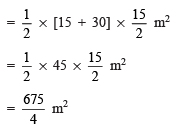∴ Area of the pentagonal shape =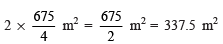Kavita’s diagram:

The given shape is splitted into a square and a triangle.

Area of the square = 15 m * 15 m = 225 m2

Area of the triangle =  1/2 * 15 * 15 =225/2 m2 = 112.5 m2

∴ Area of the pentagonal shape = 112.5 + 225 = 337.5 m2

Another way of finding the area of the pentagonal shape: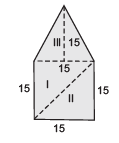By splitting the pentagonal shape into 3 triangles, we have:

Area of triangle I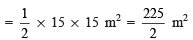Area of triangle II =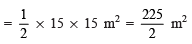Area of triangle III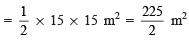∴ Area of the pentagonal shape =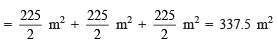Question 11. Diagram of the adjacent picture frame has outer dimensions = 24 cm ¥ 28 cm and inner dimensions 16 cm ¥ 20 cm. Find the area of each section of the frame, if the width of each section is same.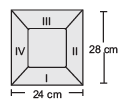Solution: Area of trapezium I:

Parallel sides are 24 cm and 16 cm.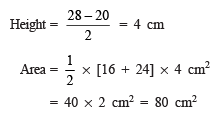Area of trapezium II:

Parallel sides are 20 cm and 28 cm.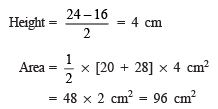Area of trapezium III:

Area of trapezium III = Area of trapezium I = 80 cm2

Area of trapezium IV:

Area of trapezium IV = Area of trapezium II = 96 cm2

Offer running on EduRev: Apply code STAYHOME200 to get INR 200 off on our premium plan EduRev Infinity!

89 docs|16 tests

,

,

,

,

,

,

,

,

,

,

,

,

,

,

,

,

,

,

,

,

,

;